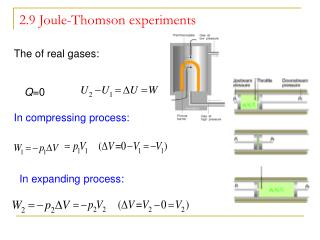# 2.9 Joule-Thomson experiments - PowerPoint PPT PresentationDownload Presentation2.9 Joule-Thomson experiments

2.9 Joule-Thomson experiments
Download Presentation## 2.9 Joule-Thomson experiments

- - - - - - - - - - - - - - - - - - - - - - - - - - - E N D - - - - - - - - - - - - - - - - - - - - - - - - - - -
##### Presentation Transcript

1. The of real gases: Q=0 In compressing process: In expanding process: 2.9 Joule-Thomson experiments

2. so Define and Joule-Thomson coefficient The enthalpy keep constant in the whole process Experimentally determined isenthalpic curve

3. He         40 K      N2         621 K      O2         764 K      Ne         231 K Inversion temperature of real gases Inversion curve μ>0, P↓, △P<0, △T<0,T ↓decrease μ =0, P↓, △P<0, △T=0,T not change μ<0, P↓, △P<0, △T>0,T increase

4. 273K, 1atm Joule-Thomson Apparatus

5. Work principle of a refrigerator Application of the Joule-Thomson effect Liquefying GASes using an isenthalpic expansion

6. Why μ>0, =0 or <0 ?

7. Discussions next class: Application of the first law • Group 1: Understanding about the atmosphere and climate phenomenon • (1) Marine climate/ Continental climate • (2) Altitude/temperature • Group 2: Is it possible water being used as fuel? • Group 3: Food and energy reserves.

8. = S S = D Q U ( - U ( U product） reactant） V r 2.9 The thermochemistry • The energy changes in chemical reactions • the heat produced or required by chemical reactions Measurable predictable At the same temperature ( reactants, products)

9. molar enthalpy of reaction Standard molar enthalpy of reaction The enthalpy change per mole for conversion of reactants in their standard states into products in their standard states, at a specified temperature and pressure Pθ H2(g,p) + I2(g,p)=2HI(g,p) △rHm(298.15K) = -51.8kJ·mol For example:

10. △rHm(298.15K) (298.15K) Calculation of standard enthalpy of reactions • (1) By standard molar enthalpy of formation The enthalpy change when one mole of the compound is formed at 100 kPa pressure and given temperature from the elements in their stable states at that pressure and temperature.

11. Cl(g)+H(g) F(g)+H(g) 300 H(g) 200 -431 +218 100 KJ.mol-1 F2 Cl2 H2 -92 -564 -100 HCl(g) -269 -200 -300 HF(g) Understanding standard molar enthalpy of formation Standard molar enthalpy of formation of the stable forms of the elements is zero; Any form of elements other than the most stable will not be zero; such as C (diamond), C (g), H (g), and S (monoclinic).

12. H2+O2 0 -50 -188 -242 -100 KJ.mol-1 -285 -150 H2O2 -200 H2O(g) -250 H2O(l) -300 The ∆fH depend on state of substances

13. C2H5OH(g) C4H6(g) H2O(g) H2(g) -235.10 110.16 -241.81 0 Example: Calculate the standard enthalpy of following reaction at 25℃ by using standard molar enthalpy of formation

14. (2) By standard molar enthalpy of combustion Definition: The enthalpy change when a mole of substance is completely burnt in oxygen at a given temperature and standard pressure. All these complete products have an enthalpy of combustion of zero.

15. Standard molar enthalpy of combustion C+O2 400 -110 CO 300 200 -394 KJ.mol-1 -284 100 CO2 0

16. （A） （B） （C） (D) 298.15K, 100kPa： CH3COOH(l)+2O2(g)=2CO2(g)+2H2O(l)

17. (3) By bond energies and bond enthalpies

18. 1/2N2(g)+3/2H2(g) NH3(g) N(g)+3H(g) 314kJ.mol-1 NH(g)+2H(g) 390kJ.mol-1 1170kJ.mol-1 1124kJ.mol-1 NH2(g)+H(g) 466kJ.mol-1 NH3(g) 1/2N2(g)+3/2H2(g) 46 kJ.mol-1

19. (4) Solublization heat

20. The experimental determination of standard enthalpy of combustion • A sample of biphenyl (C6H5)2 weighing 0.526 g was ignited in a bomb calorimeter initially at 25°C, producing a temperature rise of 1.91 K. In a separate calibration experiment, a sample of benzoic acid C6H5COOH weighing 0.825 g was ignited under identical conditions and produced a temperature rise of 1.94 K. For benzoic acid, the heat of combustion at constant pressure is known to be 3226 kJ mol–1 (that is, ΔU° = –3226 kJ mol–1.) Use this information to determine the standard enthalpy of combustion of biphenyl. ΔH = ΔU + Δ(PV) = Qv + ΔngRT

21. For example：（1） （2） （1）-（2）=（3） （3） Hess’s law The enthalpy change for any sequence of reactions that sum to the same overall reaction is identical. (Based on Enthalpy being a state function)

22. –(a)+2(b)+(c)=the above studied reaction Example 2: • C(graphite) + 2H2(g) → CH4(g) • Consider following reactions

23. Home work • Group discussion • Preview: A: 2.9 • Y: 1.12 • A: P67: 2.17(a, b) • P68: 2.38(a,b) • Y: P32: 36, 37 • P42: 45